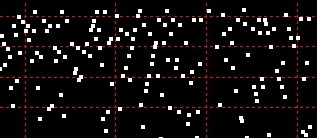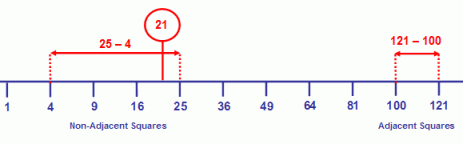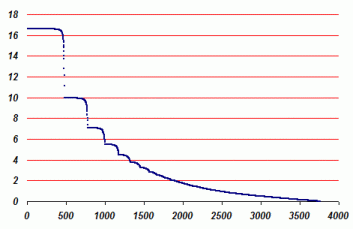exploring the undesigned
intelligence of the numberversehome   Part 2: A Deterministic Test for Compositeness Using Perfect Squares

First published March 8.
Revised June 23, 2008

What is a semiprime?
A natural number that is the product of two prime numbers. *

Using the same principle as for perfect square subtraction, the addition of perfect squares to any odd number provides a simple deterministic test for compositeness.

I am calling this a test for compositeness, rather than primality, for a couple of reasons:

• The test produces a positive proof of compositeness but a negative proof of primality (by elimination).
(... and from this it follows that...)
• It will always prove compositeness faster than primality.

The basis for a deterministic test predicated solely on the addition of perfect squares can be stated as follows:

N is composite if there exists a perfect square that when added to N creates another perfect square and providing that the two perfect squares are not adjacent.

By adjacent, I am referring to two perfect squares whose roots are in counting order (so that Ra = Rb + 1). So, for example, 25 and 36 are adjacent because their respective roots - 5 (5*5=25) and 6 (6*6=36) - are separated by 1.

As stated in the previous column, every odd number can be created by subtracting two, and only two, successive perfect squares:

In number theory, the fundamental theorem of arithmetic (or unique-prime-factorization theorem) states that every natural number greater than 1 can be written as a unique product of prime numbers.

So, for example, the unique prime factorization of 999 is: 3 * 3 * 3 * 37.

We can now state a "fundamental theorem of perfect square subtraction" such that every odd natural number is the subtractive product of two, and only two, adjacent perfect squares.

You will see that to obtain through subtraction, say, 999, you will need to subtract some relatively large perfect squares. The unique perfect square subtraction that produces 999 is: 249001- 250000.

There is a further statement we can make about composite numbers that is of great significance because we cannot make it about prime numbers:

For every composite number that is not itself a perfect square there exists a pair of nonadjacent perfect squares whose subtractive product is equal to the composite.The graphic illustrates that 21 is the subtractive product of nonadjacent squares (4 and 25)
and adjacent squares (100 and 121). Therefore it must be composite.

If you've been paying attention, you may notice a very beautiful fact - namely that for any N the square roots of the nonadjacent squares are the progenitors of its factors. If the number is a semiprime, they are the progenitors of its prime factors.

What do I mean by progenitor?

The difference between these two square roots gives one factor; the sum of these two square roots gives the other factor.

It's that simple - and it's true for every composite that's not a perfect square.

Thus, for 21, the square roots of the nonadjacent squares are sqr(4) = 2 and sqr(25) = 5.

5 - 2 = 3
5 + 2 = 7
3 * 7 = 21

How simple and satisfying is that!

If only it were easier to find the nonadjacent squares than it is to find their factors! If such a method exists, it has never been disclosed - and would, in fact, have epic consequences for the theory of complexity classes and the secure application of information technology.

If you're interested in Fermat's factorization method, read:
Fixing Fermat’s Factorization Method

• We can say with certainty that the nonadjacent perfect squares cannot be greater than the adjacent perfect squares because that would mean the subtractive product would be greater than the composite being considered.
• It would appear that, if one of the adjacent squares is close to the upper bound, the other should be close to the lower bound (that is, 1).
• There is the very important caveat that the rule of nonadjacent perfect squares does not apply to odd perfect squares. For example, the odd perfect square 9 (32) cannot be created by nonadjacent squares less than its adjacent squares (16, 25). This is a property that the odd squares share with all primes. It needs to be noted and understood; otherwise, any algorithm we attempt to construct from our observations so far will incorrectly find these odd perfect squares to be primes.

Although it would seem probable that a composite must lie within the span of its nonadjacent squares, this is only consistently the case for the first few composites. For composites greater than 55, the lower nonadjacent square can be less or more than the integer being tested. Let's consider all the odd composites under 100:

 Integer Adjacent Squares Nonadjacent Squares # of Additions Ratio (Add. / Int.) 15 49 64 1 16 1 6.67 21 100 121 4 25 2 9.52 27 169 196 9 36 3 11.11 33 256 289 16 49 4 12.12 35 289 324 1 36 1 2.86 39 361 400 25 64 5 12.82 45 484 529 4 49 2 4.44 51 625 676 49 100 7 13.73 55 729 784 9 64 3 5.45 57 784 841 64 121 8 14.04 63 961 1024 1 64 1 1.59 65 1024 1089 16 81 4 6.15 69 1156 1225 100 169 10 14.49 75 1369 1444 25 100 5 6.67 77 1444 1521 4 81 2 2.6 81 1600 1681 144 225 12 14.81 85 1764 1849 36 121 6 7.06 87 1849 1936 169 256 13 14.94 91 2025 2116 9 100 3 3.3 93 2116 2209 196 289 14 15.05 95 2209 2304 49 144 7 7.37 99 2401 2500 1 100 1 1.01

Yellow indicates the cases where the lower nonadjacent square is greater than the number being tested. The importance of where the lower nonadjacent square occurs is simply that this is the minimum number of perfect square additions required to prove compositeness.

Out of this small sample, the highest number of perfect square additions before finding a nonadjacent pair is 15% of N. It turns out, that this is fairly near the highest percentage for the composites I've investigated so far. The highest "proving ratio" for all composites under 10,000 is 6.006006....: that's 16.65%, or 1/6th of N. Samples in the 105s, 106s, and 107s are just .02% higher: 16.67%, or 5.9988.

There is reason to believe that N / ~6 must have some significance. Of all the odd composites under 10,000, 449 out of 3747 have ratios in the 16th percentile. The next largest group are 289 that have have ratios in the 9th percentile. Only 18 composites lie between these two percentiles. All other composites, about 80%, have ratios less than 9%. If you graph it (and you can do this with the downloadable data on these composites), it looks like this:Distribution of 'proving ratios' by percentagesDistribution of integers by 'proving ratios'

If you wanted to optimize the speed of the algorithm you would first focus on the percentiles that yield the most results in these charts: the 16th, the 9th, the 7th, the 5th, and so on. However, to find primes, you would still need to look at all squares up to (N / 5.9)2 to be sure.

BTW
This value of (N/5.9)2 appears to be related to the growth of the Riemann zeta function (specifically, the Lindelof hypothesis, a value that approaches 1/6th) and to the Basel problem (Euler's method for approximating Pi using infinite series, as illustrated in ZetaTest).

With N / ~6, a practical perfect square algorithm is possible, but only for numbers less than about 107. Beyond this, the geometric increase in the number of perfect square additions means that the computation time is too great. Even for numbers less than 107, perfect square addition requires many more computations than trial division, which has the great advantage of only needing to consider factors up to the square root of N. The comparison is actually worse than that because with trial division, we also get to divide SqRt(N) by 2 since we can exclude the even numbers. We can't exclude the even perfect squares - they are as important as the odd ones in this algorithm.

The arithmetic of the nonadjacent pair

For any given odd number, it would appear not much else can be predicted about its nonadjacent pair. Both members of the pair are unknown, and the relationship that may or may not exist is entirely defined by N, if N is a composite number, thus:

N = Y2 - X2

where Y2 > (X + 1)2

The following equivalences are simple arithmetic:

 Equation Root Example Square Example N = Y2 - X2 87 = 162 - 132 87 = 256 - 169 N + X2 = Y2 87 + 132 = 162 87 + 169 = 256 Y2 - N = X2 162 - 87 = 132 256 - 169 = 87

Putting the algorithm together

Having set realistic expectations for what the perfect-square-addition algorithm will be capable of doing, let's now proceed with formulating exactly what it is.

To create a test of compositeness or primality, the middle expression shown above (N + X2 = Y2), requiring addition, is an obvious choice. It only requires adding N to each perfect square from 1 to the upper bound to determine if there exists a product that is equal to another perfect square.

We know from the foregoing investigation how many X2s that N must be added to in order to determine compositeness - it's just a tad more than N / 6. In other words, to determine whether such a pair exists for an integer, we must simply add N to every perfect square from 1 through N / 5.9. If we have confidence in N / 5.9, we should also have confidence that we will find that all integers without the property of a nonadjacent square are primes - by elimination: a negative proof of primality.

Expressing what has been said as an algorithm, we can begin with stating the two necessary conditions:

If N + X2 = Y2
and
If Y - X > 1
N = Composite
Else
N = Prime

Then we need to increment the value of X for every perfect square less than N / 6. But before this we need to add the test that the number being tested is not itself a perfect square (a requirement explained above).

If Int(Sqr(N)) = Sqr(N)  'if square root is whole number
N = Composite (Perfect Square)
Exit

For X = 1 to Int(N/5.9)

If N + X^2 = Y^2
and
If Y - X > 1
N = Composite
Else
N = Prime

We may also wish to eliminate the trivial cases: all even numbers (they will fail the test anyway) and those divisible by 5 (if we choose to).

A JavaScript Implementation

The following illustrative demonstration shows that the algorithm is easy to code and effective for relatively small numbers (less than 10,000,000).

### Prove Compositeness with Perfect Squares

If N + X^2 = Y^2 and Y - X > 1 then
N = Composite Else N = Prime

Number to Test

N + Perfect Square 1 = Perfect Square 2

Iterations (# of perf. sqrs. tested)

Result

### Is the algorithm scalable?

Without finding an effective strategy for reducing the upper bound or increasing the lower bound, the number of iterations (perfect squares) becomes unmanageable beyond 107. At this point, trial division is still extremely fast - there's really no contest. The problem is there are simply a lot of perfect squares to add N to even if the upper bound holds at N / ~6. For example, a number on the order of 1012 will require at least a 150,000 iterations of the test because there are a 1,000,000 perfect squares under 100,000,000,000.

However, it does seem likely that only a discrete subset of perfect square additions have the potential to yield the desired result. This is illustrated in the following section of a matrix generated using the code disclosed in the previous column. The composites of nonadjacent perfect squares have been highlighted in orange. This is what it looks like when the table has 250 columns (or minus squares) - merely the first 250 odd numbers.You can see right away that only alternating rows participate in finding composites and the other rows are completely even. However, this should not be taken to mean that we only need to test odd perfect squares. If you do this - say, by changing the JavaScript for loop from for (cps = 1; cps <= max; cps++) to for (cps = 1; cps <= (max-1); cps=cps+2) - you will get false prime results for many composites.

The reason for this lies in the stepping of the vertical perfect squares. They are alternating odd and even, but you don't see them in the generated worksheet. You have to recall the matrix presented in Part 1. Here, I've highlighted the odd numbers in yellow:

 PS PS-1 PS PS-4 PS PS-9 PS PS-16 PS P-25 PS PS-36 PS PS-49 4 3 9 5 16 7 25 9 36 11 49 13 64 15 9 8 16 12 25 16 36 20 49 24 64 28 81 32 16 15 25 21 36 27 49 33 64 39 81 45 100 51 25 24 36 32 49 40 64 48 81 56 100 64 121 72 36 35 49 45 64 55 81 65 100 75 121 85 144 95 49 48 64 60 81 72 100 84 121 96 144 108 169 120 64 63 81 77 100 91 121 105 144 119 169 133 196 147 81 80 100 96 121 112 144 128 169 144 196 160 225 176 100 99 121 117 144 135 169 153 196 171 225 189 256 207

There are other levels of organization in the matrix that need to be studied and might yield improvements in the algorithm. These improvements could possibly produce a far more efficient probabilistic test. However, for now, the perfect square compositeness test has a long way to go before it can rival probabilistic tests (based on FLT) or deterministic tests (based on trial division)..... Your suggestions are welcome!